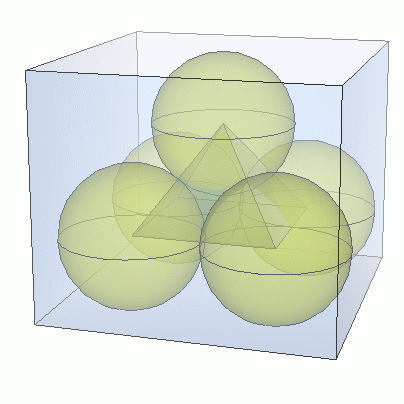SEARCH HOMEMath Central Quandaries & QueriesQuestion from jasmin, a student: Four spherical balls having diameter of 4 cm are placed in a square box whose inside base dimensions are 8 cm. In the space between the first four spherical balls, a fifth spherical ball of the same diameter is placed. How deep must the box be in order that the top will touch the fifth ball?Hi Jasmin.

This just takes a little bit of geometry. Here's a hint: Draw the shape connecting all five centers of the spheres:You get a square-based pyramid in which all edges have the same length: two radii, so 4 cm.

If you can determine the height of the pyramid, you can easily see the height the box needs to be.

Work out the pyramid's height by using Pythagoras' Theorem twice.

Hope this helps,
Stephen La Rocque.Math Central is supported by the University of Regina and The Pacific Institute for the Mathematical Sciences.